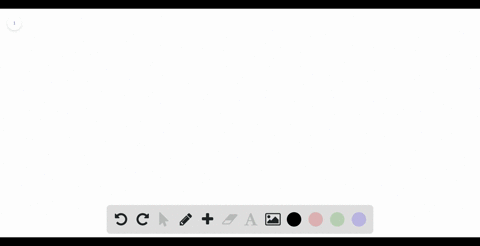### Use Definition 2 to find an expression for the ar…

00:47University of California, Berkeley
Problem 21

Use Definition 2 to find an expression for the area under the graph of $f$ as a limit. Do not evaluate the limit.

$f(x) = \dfrac{2x}{x^2 + 1}, \hspace{5mm} 1 \le x \le 3$

$\lim _{n \rightarrow \infty} \sum_{i=1}^{n}\left[\frac{2\left(1+\frac{2 i}{n}\right)}{\left(1+\frac{2 i}{n}\right)^{2}+1} \cdot \frac{2}{n}\right]$

## Discussion

You must be signed in to discuss.

## Video Transcript

Okay. The first thing we know is that Delta Axe is three minus one over ad, which is two over. And we know acts of eyes one plus items to over. And therefore, what we know is that we have the limit as UN approaches infinity starting at one like this.